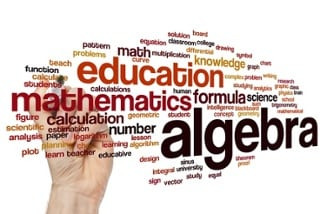Select Page

# 4 Common Misconceptions Students Have About AlgebraIn the United States, algebra is seen as the gateway course to higher-level math courses and, in some cases, even college. This is oftentimes a student’s first encounter with abstract mathematical thinking, which leads to confusion and uncertainty. Here are four common misconceptions students of all ages have about algebra:

1)      Assuming algebra makes math harder

Many students arrive to their first algebra class with the preconceived notion that algebra is a difficult subject that is unrelated to any math they have done in the past. The name alone is daunting. What these students may not realize is that they have been thinking algebraically for quite some time—recognizing and describing patterns, using inverse operations, and finding unknown values. The main difference is that true algebra integrates the use of variables in accomplishing these tasks to make math more versatile. Making students aware of these similarities and deliberately connecting this new notation to previously learned concepts can help eliminate some of the anxiety that students initially bring into the classroom.

2)      Confusing the variable x with the operation xBecause of their exposure to mathematical operations in elementary math, pre-algebra students recognize “x” in a problem as an indication to multiply two numbers together. Once they reach algebra, x is introduced as a variable. Students may wonder, “Why does the italicized letter suddenly mean something different?” This becomes especially confusing when solving algebraic problems by hand. Since there is no stylized difference between x as a variable and x as an operation, some students may misinterpret the variable x to indicate multiplication with the following term. Be sure to emphasize that parentheses or the symbol “∙” are used to indicate multiplication in upper-level mathematics.

3)      Combining non-like terms

One of the most common misconceptions for students brand new to algebra is the idea that 5x + 4 equals 9x. It is understandable how students might simplify this expression based on their prior knowledge of adding integers, but once again, they fail to recognize x as a variable term. Since the second term only has a numeric part, 5x and 4 are not like terms, and so they cannot be combined into a single term. Even higher-level algebra students make this mistake—it is not uncommon to see the expression 3x2 + 4x3 simplified to 7x5 rather than recognizing that these terms cannot be combined since they have different exponents. Asking students to substitute a random value in for x, so they can see that these expressions are not equivalent, is one way to teach that non-like terms cannot be combined.

4)      Interpreting variables as constantsWhen students first start solving algebraic equations, it may be difficult for some to grasp that a variable is actually variable. Just because you determine x = 3 for one particular equation does not mean that x = 3 for all equations involving an x. Oftentimes, this results from a lack of understanding that the x itself merely represents an unknown value that can be determined using the other information in the equation. This misconception is further highlighted when examining equations that contain more than one variable. For example, if x + y = 7, x equals 5 when y equals 2. However, this is not the only solution—there are an infinite number of solutions for x and y. This can be demonstrated by graphing a linear function that will show the solution not to be a singular point.

You can learn why x usually represents the unknown from the TED talk by Terry Moore. Finding ways to help students better understand the concept of a variable and its applications can alleviate many algebraic misconceptions.

# Subscribe to our blog!

Stay up-to-date on the latest Higher Ed, K-12 and Curriculum Design content.